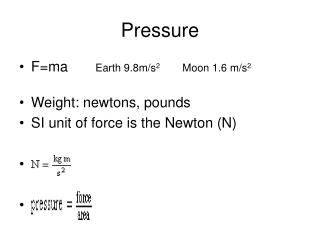DownloadDownload PresentationPressure

# Pressure

Télécharger la présentation## Pressure

- - - - - - - - - - - - - - - - - - - - - - - - - - - E N D - - - - - - - - - - - - - - - - - - - - - - - - - - -
##### Presentation Transcript

1. Pressure • F=ma Earth 9.8m/s2 Moon 1.6 m/s2 • Weight: newtons, pounds • SI unit of force is the Newton (N)

2. Pressure Pressure is a measure of force per unit area. Units for pressure include: atmospheres (atm), pascals (Pa), kilopascals (kPa), mm Mercury (mm Hg), and torr.

3. Pressure unit A pressure unit that is equivalent to the pressure at sea-level. 1 atmosphere (atm) = 101.325 kPa = 101,325 Pa = 760 torr = 760 mm Hg.

4. Kilopascal (kPa) • The kilopascal is a commonly used unit of pressure that is equal to 1000 Pa. • The kilopascal is more commonly used than the pascal because the pascal is such a small unit of pressure. • 101.325 kPa = 101,325 Pa = 1 atmosphere (atm) = 760 torr = 760 mm Hg.

5. Molecular motion in a gas The molecules in a gas are in constant motion. The purple balls represent gaseous atoms that collide with each other and the walls of the container. Gas molecules are in constant motion. "Pressure" is a measure of the collisions of the atoms with the container

6. Collisions Pressure is proportional to the collision frequency of molecules against the walls of the container.

7. Pressure determines the direction of mass flow

8. Dalton's Law of Partial Pressures: The total pressure exerted by a mixture of gases equals the sum of the partial pressures of the individual gases.   P (total) = P1 + P2 + P3 + ...

9. The water column is on the inside: PATM = PGAS + PWV + PWC

10. There is no water column: PATM = PGAS + PWV

11. The water column is on the  outside: PATM + PWC   = PGAS + PWV